# Impedance

While Ohm's Law applies directly to resistors in DC or in AC circuits, the form of the current-voltage relationship in AC circuits in general is modified to the form:where I and V are the rms or "effective" values. The quantity Z is called impedance. For a pure resistor, Z = R. Because the phase affects the impedance and because the contributions of capacitors and inductors differ in phase from resistive components by 90 degrees, a process like vector addition (phasors) is used to develop expressions for impedance. More general is the complex impedance method.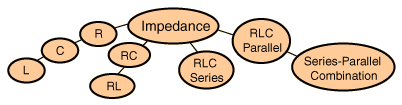Series and parallel combination of any two impedances
Index

AC circuit concepts

 HyperPhysics***** Electricity and Magnetism R Nave
Go Back

# Impedance Combinations

Combining impedances has similarities to the combining of resistors, but the phase relationships make it practically necessary to use the complex impedance method for carrying out the operations. Combining series impedances is straightforward: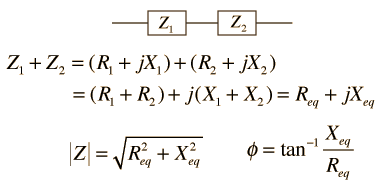Calculation

Combining parallel impedances is more difficult and shows the power of the complex impedance approach. The expressions must be rationalized and are lengthy algebraic forms.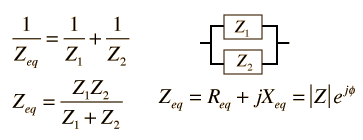Expressions
Index

AC circuit concepts

 HyperPhysics***** Electricity and Magnetism R Nave
Go Back

# Parallel Impedance Expressions

The complex impedance of the parallel circuit takes the form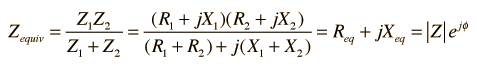when rationalized, and the components have the form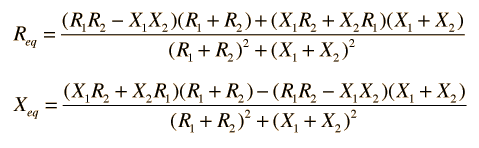Calculation
Index

AC circuit concepts

 HyperPhysics***** Electricity and Magnetism R Nave
Go Back

# Impedance Calculation

Impedances may be combined using the complex impedance method.

For
Z1 = + j
Z2 = + j

the series combination is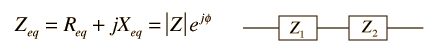Zeq = + j= at phase.

The parallel combination is

 Zeq = + j= at phase.The units for all quantities are ohms. A negative phase angle implies that the impedance is capacitive, and a positive phase angle implies net inductive behavior.

 Parallel impedance expressions Inductive reactance Capacitive reactance
Index

AC circuit concepts

 HyperPhysics***** Electricity and Magnetism R Nave
Go Back